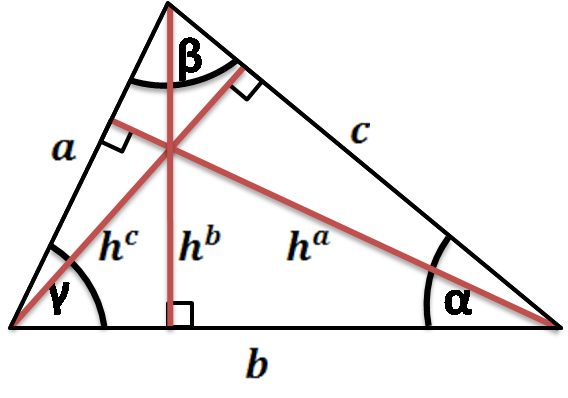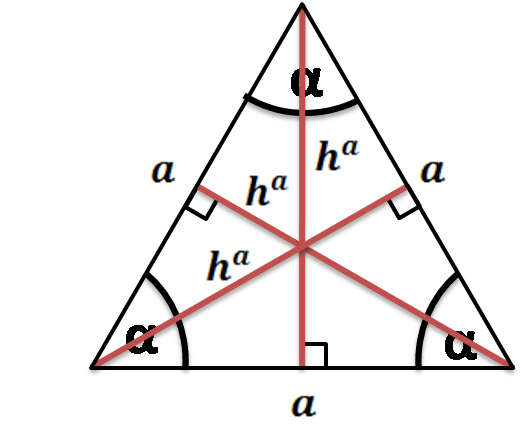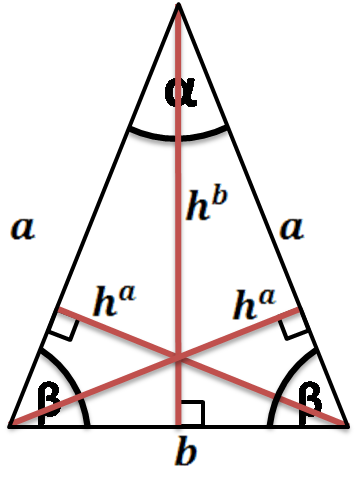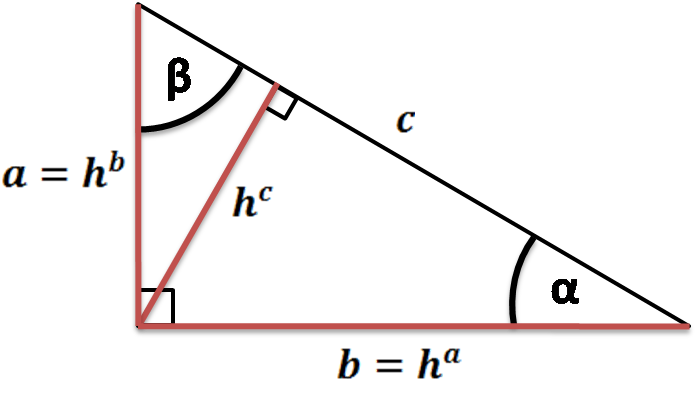# Triangle Height Calculator

Created by Hanna Pamuła, PhD candidate
Reviewed by Bogna Szyk
Last updated: Sep 27, 2022

If you are looking for an easy tool to calculate the height in any triangle, you're in the right place - this triangle height calculator is the tool for you. Whether you are looking for the triangle height formulas for special triangles such as the right, equilateral or isosceles triangle or any scalene triangle, this calculator is a safe bet - it can calculate the heights of the triangle, as well as triangle sides, angles, perimeter, and area. Don't wait any longer; give it a go!

If you are still wondering about the formula for the height of an equilateral triangle or how to find height without area, keep scrolling, and you'll find the answer.

## What is the altitude of a triangle?

Every side of the triangle can be a base, and from every vertex, you can draw the line perpendicular to a line containing the base - that's the height of the triangle. Every triangle has three heights, which are also called altitudes. Drawing the height is known as dropping the altitude at that vertex.## How to find the height of a triangle - formulas

There are many ways to find the height of the triangle. The most popular one is the one using triangle area, but many other formulas exist:

1. Given triangle area

The well-known equation for the area of a triangle may be transformed into a formula for the altitude of a right triangle:

• area = b × h / 2, where b is a base, h - height
• so h = 2 × area / b

But how do you find the height of a triangle without area? The most popular formulas are:

1. Given triangle sides

It's using an equation called Heron's formula that lets you calculate the area, given sides of the triangle. Then, once you know the area, you can use the basic equation to find out what is the altitude of a triangle:

• Heron's formula: area = 0.25 × √((a + b + c) × (-a + b + c) × (a - b + c) × (a + b - c))
• so h = 0.5 × √((a + b + c) × (-a + b + c) × (a - b + c) × (a + b - c)) / b

1. Given two sides and the angle between

Use trigonometry or another formula for the area of a triangle:

• area = 0.5 × a × b × sin(γ)

(or area = 0.5 × a × c × sin(β) or area = 0.5 × b × c × sin(α) if you have different sides given)

• h = 2 × 0.5 × a × b × sin(γ) / b = a × sin(γ)

If your shape is a special triangle type, scroll down to find the triangle height formulas. Simplified versions of the general equations are easier to remember and calculate.

## How to find the height of an equilateral triangle

An equilateral triangle is a triangle with all three sides equal and all three angles equal to 60°. All three heights have the same length that may be calculated from:

• h△ = a × √3 / 2, where a is a side of the triangleIn an equilateral triangle, the altitudes, the angle bisectors, the perpendicular bisectors, and the medians coincide.

If you're interested in the formulas for the area and perimeter, visit our dedicated equilateral triangle calculator.

## How to find the height of an isosceles triangle

An isosceles triangle is a triangle with two sides of equal length. There are two different heights of an isosceles triangle; the formula for the one from the apex is:

• hᵇ = √(a² - (0.5 × b)²), where a is a leg of the triangle, and b is a base. The formula is derived from the Pythagorean theorem.

• The heights from base vertices may be calculated from e.g.

• area formula: hᵃ = 2 × area / a = √(a² - (0.5 × b)²) × b / a
• trigonometry: hᵃ = b × sin(β)For the area and perimeter formulas of this type of triangle, visit our dedicated isosceles triangle calculator.

## How to find the altitude of a right triangle

A right triangle is a triangle with one angle equal to 90°. Two heights are easy to find, as the legs are perpendicular: if the shorter leg is a base, then the longer leg is the altitude (and the other way round). The third altitude of a triangle may be calculated from the formula:

• hᶜ = area × 2 / c = a × b / cIf you're interested in the area and perimeter equations of this triangle, look at our right triangle calculator.

## How to find the altitude of the triangle with this triangle height calculator?

After reading our explanation, we're pretty sure that now you understand how to find the height of a triangle without the area given or what is the altitude of a triangle. But let's look at a simple example to show you the flexibility of our tool:

1. Choose the triangle type. Assume we want to calculate the heights of a scalene triangle, so we don't change the default option.
2. Enter the given values. It could be three sides or two sides and an angle. Let's stay with the first option: a = 6 in, b = 14 in, c = 17 in.
3. The triangle height calculator displayed all three heights - they are equal to 13.17 in, 5.644 in, and 4.648 in. What is more, the calculator showed us all triangle angles, area, and perimeter. That's awesome!
Hanna Pamuła, PhD candidate
Special triangle?
noSides
a
in
b
in
c
in
Heights (altitudes)
hᵃ
in
hᵇ
in
hᶜ
in
Angles
α
deg
β
deg
γ
deg
Others
Area
in²
Perimeter
in
People also viewed…

### Black Hole Collision Calculator

The Black Hole Collision Calculator lets you see the effects of a black hole collision, as well as revealing some of the mysteries of black holes, come on in and enjoy!

### Cholesky decomposition

The Cholesky decomposition calculator lets you quickly and easily obtain the lower triangular matrix of the Cholesky factorization. Pick between a 2×2, 3×3, or a 4×4 matrix.

### Modulo of negative numbers

We teach you how to understand the modulo operator of negative numbers.

### Titration

Use our titration calculator to determine the molarity of your solution.